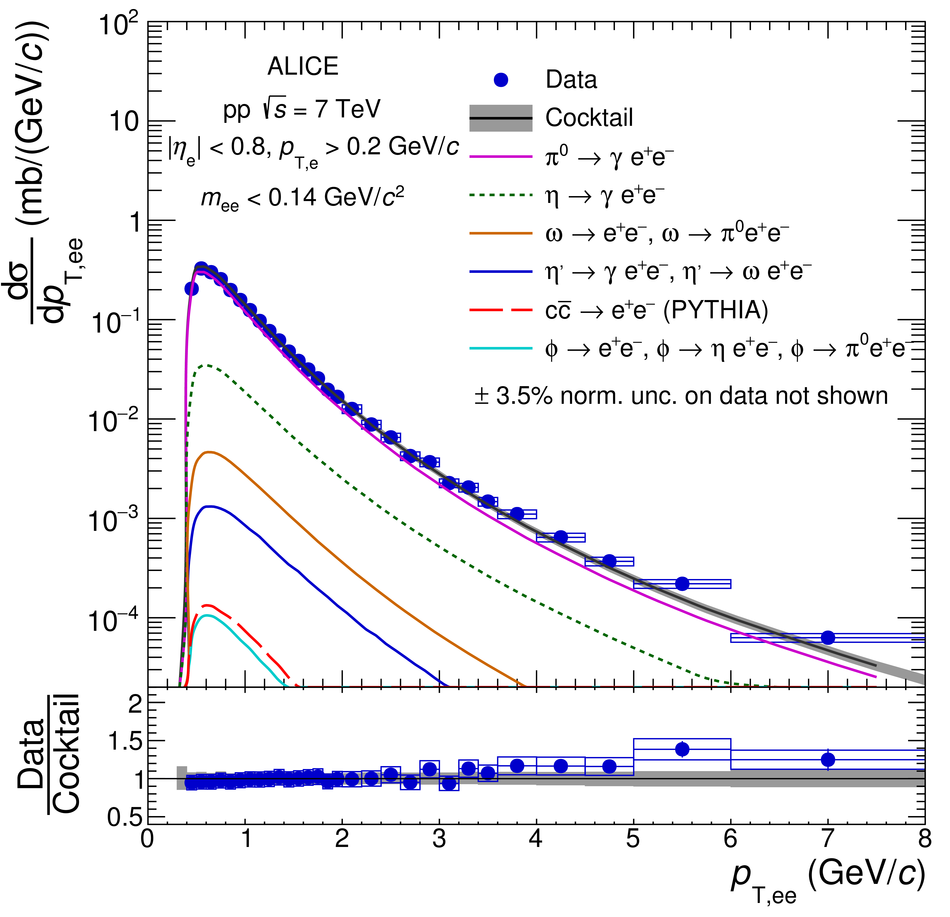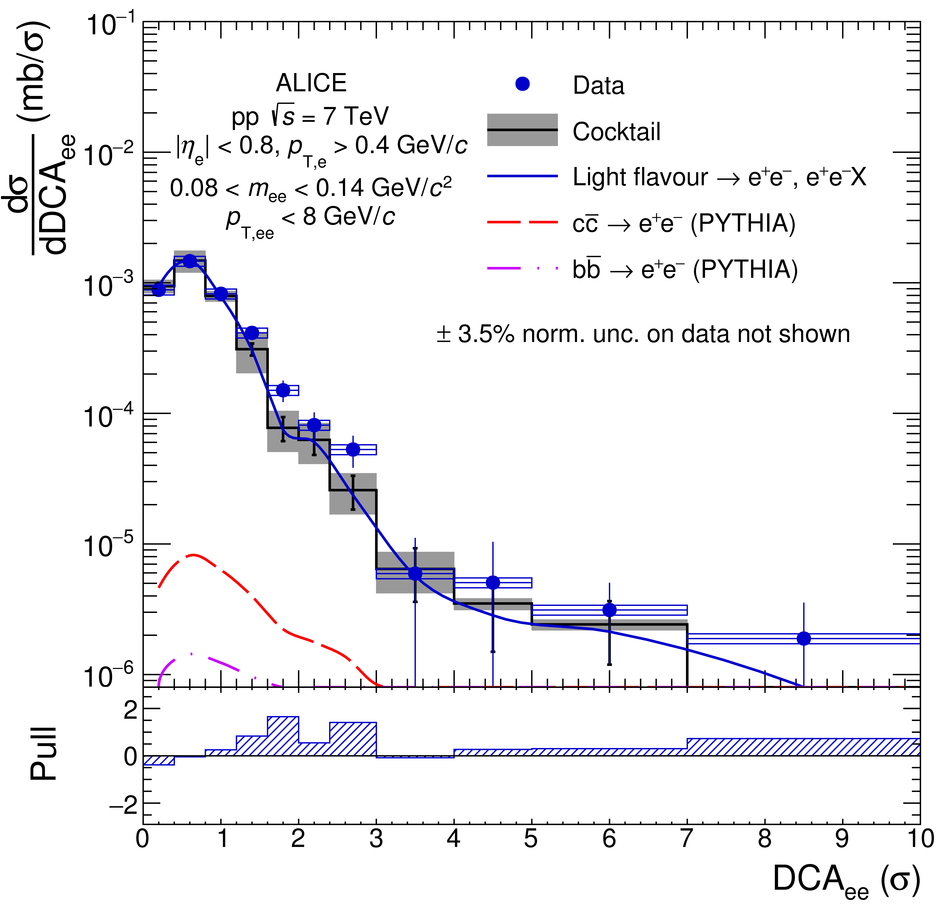Figure 10

 Inclusive \ee cross section in \pp collisions at \roots = 7\,TeV in the ALICE acceptance as a function of \ptee (left) and \dcaee (right) for \mee $<$ 0.14~\GeVovercs and 0.08 $<$ \mee $<$ 0.14~GeV/$c^{2}$, respectively. The data are compared with a cocktail of expected sources. In the bottom panels, the ratio of data to cocktail as a function of \ptee (left) and the pull distribution as a function of \dcaee (right) are shown. Statistical and systematic uncertainties on the data are plotted as vertical bars and boxes, respectively. The total uncertainty of the cocktail is represented as a grey band.